# Atoms and the Periodic tablePage 4

#### WATCH ALL SLIDES

Slide 29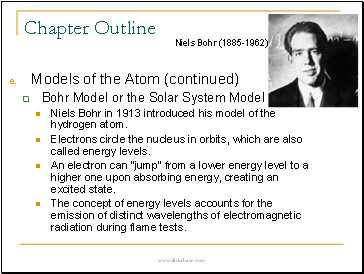Chapter Outline

• Models of the Atom (continued)

Bohr Model or the Solar System Model

Niels Bohr in 1913 introduced his model of the hydrogen atom.

Electrons circle the nucleus in orbits, which are also called energy levels.

An electron can “jump” from a lower energy level to a higher one upon absorbing energy, creating an excited state.

The concept of energy levels accounts for the emission of distinct wavelengths of electromagnetic radiation during flame tests.

Slide 30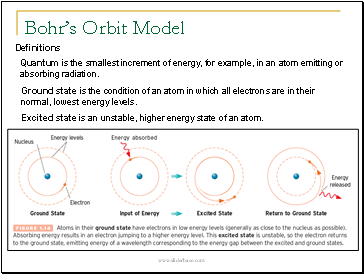Bohr’s Orbit Model

Definitions

Quantum is the smallest increment of energy, for example, in an atom emitting or absorbing radiation.

Ground state is the condition of an atom in which all electrons are in their normal, lowest energy levels.

Excited state is an unstable, higher energy state of an atom.

Slide 31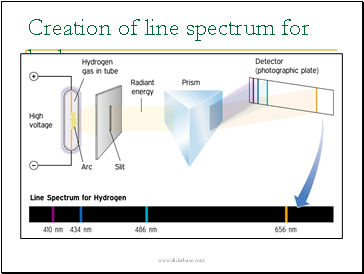Creation of line spectrum for hydrogen

Slide 32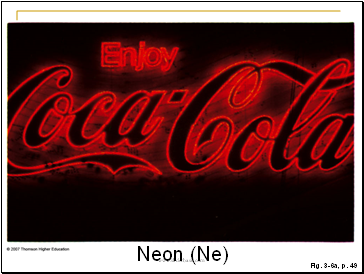Figure 3.6: Neon.

(a) A partially evacuated tube that contains neon gas gives a reddish-orange glow when high voltage is applied.

Slide 33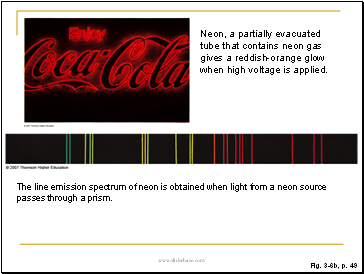Neon, a partially evacuated tube that contains neon gas gives a reddish-orange glow when high voltage is applied.

The line emission spectrum of neon is obtained when light from a neon source passes through a prism.

Slide 34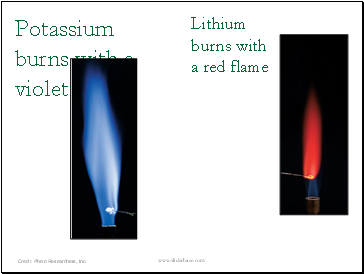Potassium burns with a violet flame

Lithium burns with a red flame

Slide 35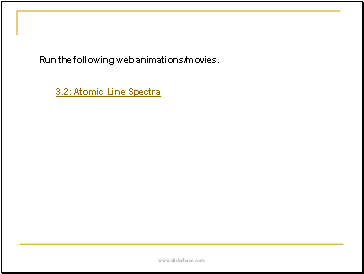## Atomic Line Spectra

Run the following web animations/movies.

3.2:

Slide 36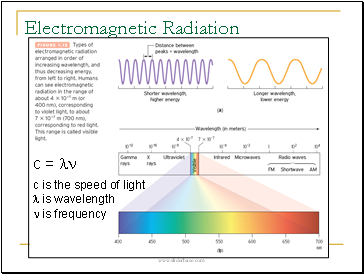Slide 37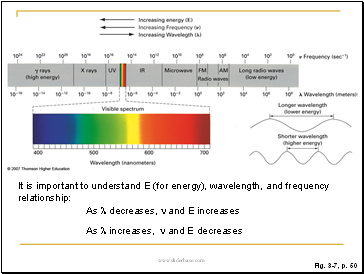Figure 3.7: The electromagnetic spectrum.

Visible light (enlarged section) is but a small part of the entire spectrum. The energy of electromagnetic radiation increases from the radio wave end to the gamma ray end. The frequency of electromagnetic radiation is related to the wavelength by vλ = c where v = frequency; λ = wavelength; and c = speed of light, 3.00 x 108 meters (m)/second (s). The higher the frequency, the lower the wavelength and the larger the energy. The energy (E) of a photon (or quantum) of light is given by the expression Ephoton = hv where h is Planck’s constant (6.6262 x 10−34 J · s).

Go to page:
1  2  3  4  5  6  7  8  9  10